# Basic functions - math word problems

1. To improve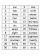To improve her handwriting Paula practices writing the numbers 1 to 200 in words. How many times will she have written the word "one" in all?
2. Symmetry by plane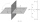Determine the coordinates of a image of point A (3, -4, -6) at a symmetry that is determined by the plane x-y-4z-13 = 0
3. Profit gainIf 5% more is gained by selling an article for Rs. 350 than by selling it for Rs. 340, the cost of the article is:
4. BucketThe bucket half filled with water weighs 5.55 kg, the full bucket weighs 9.85 kg. How much does the bucket weigh?
5. Precious metals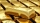In 2006-2009, the value of precious metals changed rapidly. The data in the following table represent the total rate of return (in percentage) for platinum, gold, an silver from 2006 through 2009: Year Platinum Gold Silver 2009 62.7 25.0 56.8 2008 -41.3 4
6. Young mathematician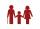One young mathematician was bored again. He found that the average age of people in the room where the seminar is equal to its count. Then his 29-year-old brother entered this room. Even then, the average age of all present was the same as the count of peo
7. Circus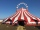On the circus performance was 150 people. Men were 10 less than women and children 50 more than adults. How many children were in the circus?
8. InqualitySolve inequality: 3x + 6 > 14
9. No smoke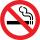Tobacco company NO-SMOKE adorned its stand at the cigarette-type trade fair with the cigarette-shaped. The dimensions of which were 20 times the size of a regular cigarette. The regular cigarette contains 0.8 mg of nicotine. How much nicotine would a giant
10. Reminder and quotientThere are given numbers A = 135, B = 315. Find the smallest natural number R greater than 1 so that the proportions R:A, R:B are with the remainder 1.
11. Balloon and bridgeFrom the balloon, which is 92 m above the bridge, one end of the bridge is seen at a depth angle of 37° and the second end at depth angle 30° 30 '. Calculate the length of the bridge.
12. Cows and calves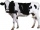There are 168 cows and calves in the cowshed. Cows are in nine stalls and calves in four stalls. Same count cows are in every cow stall and three more in each calf stall than in a cow stall. What is the capacity of the stalls for cows and what for calves?
13. Hyperbola equationFind the hyperbola equation with the center of S [0; 0], passing through the points: A [5; 3] B [8; -10]
14. Curve and lineThe equation of a curve C is y=2x² -8x+9 and the equation of a line L is x+ y=3 (1) Find the x co-ordinates of the points of intersection of L and C. (2) Show that one of these points is also the stationary point of C?A radioactive material loses 10% of its mass each year. What proportion will be left there after n=6 years?
16. Shopping center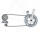The shopping center buys from the manufacturer bikes at a purchase price of 180 €. It sells them for a sale price of 250 €. However, in the advertising for the sale of these goods, the shopping center spent 20% of the selling price of all bicycles in stock
17. Simplify 3Simplify mixed numerals expression: 8 1/4- 3 2/5 - (2 1/3 - 1/4) Show your solution.
18. Winery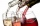The wine processing plant sold 8/15 of its total wine production to the domestic market, 2/7 to the foreign the rest of the wine. How many percents are still in stock?
19. Discount saleAt Christmas Sale after a 20% discount cosmetic package was priced at a price of 5 euros and 60 cents; later it went to 3 euros and 80 cents. How much percentage is the total discount?
20. Poplar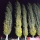How tall is a poplar by the river, if we know that 1/5 of its total height is a trunk, 1/10th of the height is the root and 35m from the trunk to the top of the poplar?

Do you have an interesting mathematical word problem that you can't solve it? Enter it, and we can try to solve it.

To this e-mail address, we will reply solution; solved examples are also published here. Please enter the e-mail correctly and check whether you don't have a full mailbox.

Please do not submit problems from current active competitions such as Mathematical Olympiad, correspondence seminars etc...